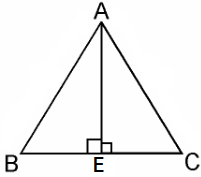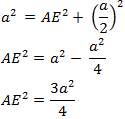Guru

# In an equilateral triangle ABC, D is a point on side BC such that BD = 1/3BC. Prove that 9AD2 = 7AB2. Q.15

• 0

Give the best and easy way to solve the problem of class 10th ncert of triangles chapter of exercise 6.5 of question no.15, it is very important question of class 10th give the best way to solve this problem In an equilateral triangle ABC, D is a point on side BC such that BD = 1/3BC. Prove that 9AD2 = 7AB2.

Share

1. Given, an equilateral triangle say ABC,Let the sides of the equilateral triangle be of length a, and AE be the altitude of ΔABC.

∴ BE = EC = BC/2 = a/2

In ΔABE, by Pythagoras Theorem, we get

AB2 = AE2 + BE24AE2 = 3a2

⇒ 4 × (Square of altitude) = 3 × (Square of one side)

Hence, proved.

• 0